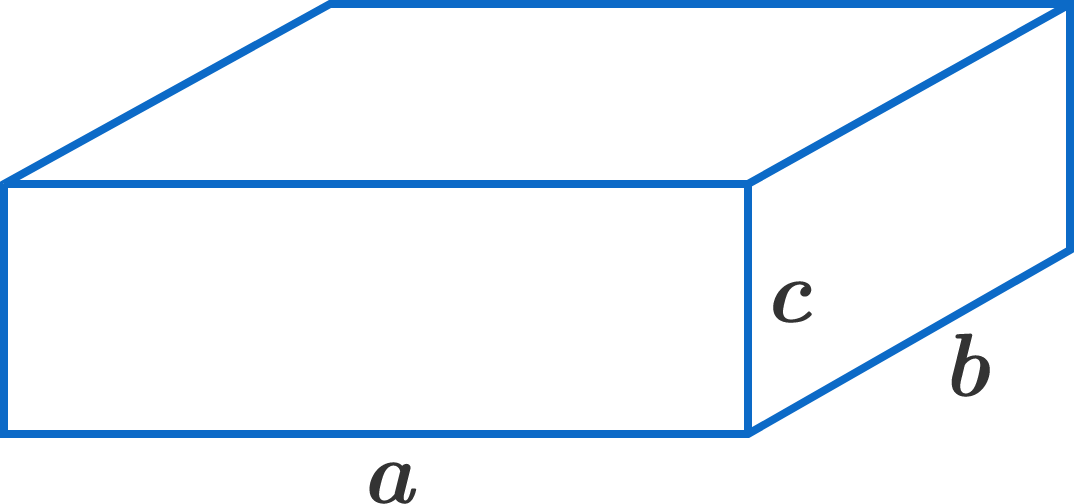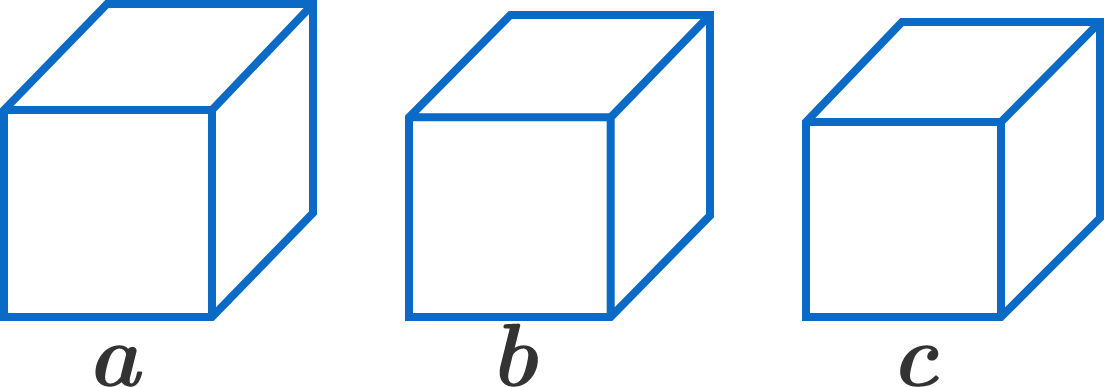# The Cuboid must be a Cube?

Geometry Level 1

True or False?

For positive reals $a,$ $b,$ and $c,$ if $3abc = a^3 + b^3 + c^3,$ then it is necessarily true that $a=b=c.$


Note: One can interpret this geometrically. Consider a cuboid with side lengths $a,b,c,$ and three cubes with respective side lengths $a,b,c.$ If the sum of the volumes of the cubes is the same as three times the volume of the cuboid, then is the cuboid necessarily a cube?×

Problem Loading...

Note Loading...

Set Loading...